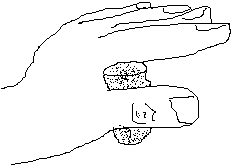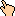## Puzzles to Come

Here are some puzzles that will hopefully grace the Brain Candy page someday:
• Shuffling cards (split & zip [interleave]) and determining how many shuffles until the order repeats
• Baseball puzzle (puzzles:125)
• Puzzles and/or games from/with Michael Arnold (puzzles:121,122)
• Jordan's puzzle page
• Lukas' combinatorial puzzle (puzzles:114)
• Ross' blood puzzle (puzzles:113)
• Jacob's moving dot puzzle (puzzles:109)
• Knight puzzles (puzzles:108)
• 10 pairs of socks puzzle
• Wine cork puzzle (courtesy Jacob Corbin)
• Take two wine corks, place one in each nook of your thumbs, as in this image:• With the opposite hand, grab the tops and the bottom of both corks, and pull your two hands apart.
• Memorization games (puzzles:103)
• Classical lateral thinking puzzle (puzzles:83)
• Matt Austern odd but not peculiar puzzle. (puzzles:101)
• The 1,1 1,2 1,1 2 1 1,1 1 1 2 2 1,etc. puzzles
• The Missing Square puzzle
• The Circle puzzle (puzzles:71)
• Cut a square into five equal pieces
• Guess 1-12, I tell you {yep,close meaning |guess-ans|<=3,not close} - how many guesses to guarantee?
• y=x/(x+1) f(0)=0 f( )=1 This is the probability you keep the amount in the envelope if the amount is only a positive number. If it's negative, then y=.5x/(|x|+1) + .5
• Worm your way through a rubiks cube and end up in the center
• Golden rope puzzle (puzzles:65)
• Max # of colors to color 2D plane of quarters
• Lower bound on separating all n chocolates in arbitrary rectangular configuration axb [n-1]
• Two fuses, each non-uniformly burning time of 1 hour. Use them to measure the following times: 45, 40, 29, (all numbers from 1-66)
• Using two pointers, determine if a linked list has a cycle.
• Three 5s and a 1 make 26
• Steve Fink's 'ho' puzzle (puzzles:54)
• Four 7s and a 1 make 100
• Josh's Dad's triangle puzzle (in which you evolve toward equilaterals)
• Tao's number-on-the-head-of-three-people puzzle
• The square of any 25,35,45,55 = tens*(tens+1)+25 [example 35 = 25 + 3*4*100 = 1225]
• More number puzzles (divisibility by 9, 11, 3, etc)
• The chess puzzles from Jordan (puzzles:50)
• The boy/girl puzzles (puzzles:49)
• The two three-person Tao/Benn logic ones (puzzles:47)
• The 6-puzzle from David Gibson (puzzles:43)
• The Geometry Puzzle from Justin (puzzles:36)
• The recursive shrunken map
• Count 1s: The largest number s.t. the # of 1s from 1 to that number (when written out) equals that number.
• The chance an integer has a 1
• The x,y stamps and the largest postage you can't make
• 2-person 1,2,5,10 river crossing in 19 (easy) and 17 (hard) minutes. Follow up with understanding when the 19-second shunting solution is optimal and when the 17-second solution is optimal. Then ask (open question) whether there are any other solutions for possible values of x1, x2, x3 and x4 (which here are assigned to 1,2,5 and 10) and if not prove why not. Also generalize for more people and more people allowed on the bridge at once (again, open question).
• Cork problem - put 2 corks in thumbs and forefingers and place them in the opposite hands thumb/forefinger crevice without letting go.
• Birthday cake (from Jordan Smith)
• Sheep and Lions (from Jordan Smith)
• Floor Tiles (from Continental)
• The cookies (from Continental)

WWW Maven: Dan Garcia (ddgarcia@cs.berkeley.edu)Send me feedback